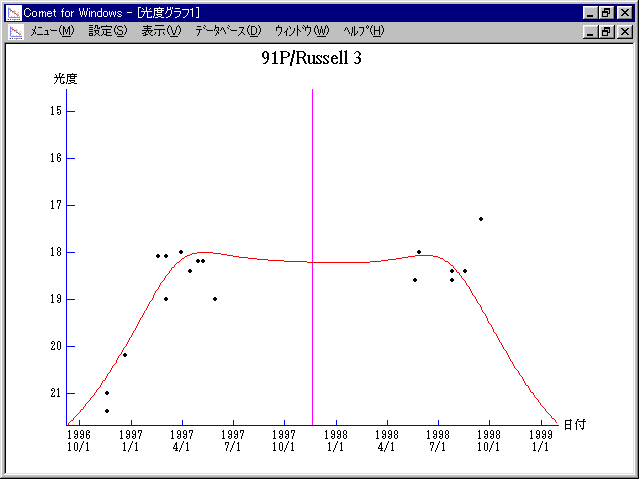\$B%i%C%;%kBh#3WB@1(B

91P/Russell 3 (1997)\$B50F;MWAG(B

The following improved orbital elements by Kenji Muraoka,
are from 99 observations 1983 to 1998, including 9 Planets,
Moon and 5 minor planets perturbations and non-gravitational
effect of style II.  The mean residual is +/- 0.79 arc seconds.

Epoch  =  1997 Nov.  8.0  TT       JDT = 2450760.5
T  =  1997 Nov. 19.21084       +/- 0.00293 (m.e.) TT
Peri. =  353.42862                +/- 0.00069
Node  =  248.67169                +/- 0.00013   (2000.0)
Incl. =   14.09576                +/- 0.00002
q  =    2.5100681              +/- 0.0000017 AU
e  =    0.3440937              +/- 0.0000003
a  =    3.8268699              +/- 0.0000008 AU
n  =    0.13165535             +/- 0.00000004
P  =    7.486                  +/- 0.0000023  years
A1  =   +5.870                  +/- 0.789
A2  =   +0.54725                +/- 0.00926\$B@1?^(B1997\$BG/(B 1\$B7n(B 2\$BF|!A(B1998\$BG/(B12\$B7n(B23\$BF|(B\$B8wEYJQ2=(B

m1 = 7.5 + 5 log\$B&\$(B + 20.0 log r\$B50F;MWAG\$OB<2,7r<#;a\$N7W;;\$K\$h\$k\$b\$N\$G\$9!#(B \$B@1?^\$O(B StellaNavigator Ver.2.0 for Windows (\$B%"%9%H%m%"!<%D(B \$BJTCx(B / \$B%"%9%-!<=PHG6I4)(B) \$B\$G:n@.\$7\$?\$b\$N\$G\$9!#(B \$B8wEY%0%i%U\$O(BComet for Windows\$B\$G:n@.\$7\$?\$b\$N\$G\$9!#(B